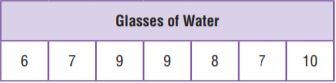Homework Explained - Math Practice 101Dear guest, you are not a registered member. As a guest, you only have read-only access to our books, tests and other practice materials.

As a registered member you can:

Registration is free and doesn't require any type of payment information. Click here to Register.
Go to page:

• Question 6

The data set shows the number of glasses of water Dalia drinks each day for a week.Part A

What is the mean number of glasses of water Dalia drinks each day?

•  glasses
• Question 6

Part B

What is the mean absolute deviation of the number of glasses of water Dalia drinks each day? Round your answer to the nearest tenth. Use words and numbers to support your answer.

• Question 7

The numbers of emails Megan received each hour are 9, 10, 9, 8, 7, and 2. The mean of the data values is 7.5 and the median is 8.5. Which measure of center better describes the data, the mean or median? Use words and numbers to support your answer.

• Type below:
• Question 8

The number of miles Madelyn drove between stops was 182, 180, 181, 184, 228, and 185. Which measure of center best describes the data?

• mean
• median
• mode

Yes, email page to my online tutor. (if you didn't add a tutor yet, you can add one here)